## Solve the system, or show that it has no solution. (If there is no solution, enter NO SOLUTION. If there are an infinite number of solutions

Question

Solve the system, or show that it has no solution. (If there is no solution, enter NO SOLUTION. If there are an infinite number of solutions, enter the general solution in terms of x, where x is any real number.)
20x − 80y = 100
−14x + 56y = −70
(x, y) =

in progress 0
6 months 2021-07-25T03:10:38+00:00 1 Answers 9 views 0

The system has an infinite set of solutions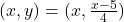Step-by-step explanation:

From the first equation: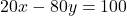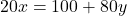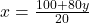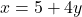Replacing on the second equation: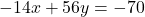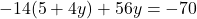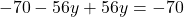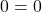This means that the system has an infinite number of solutions, considering: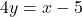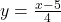The system has an infinite set of solutions